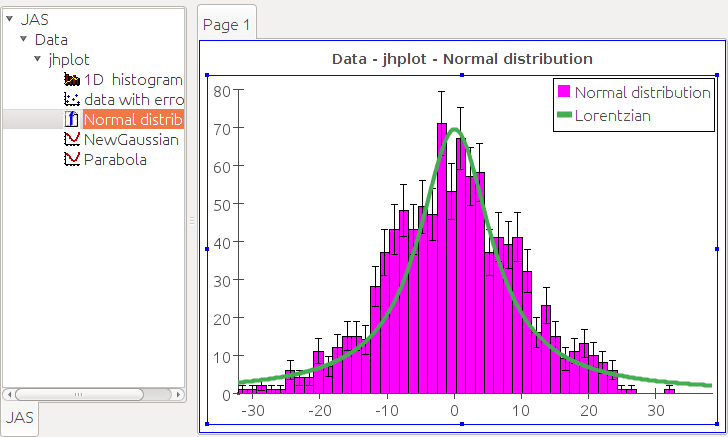# DMelt:DataAnalysis/5 Fitting Data

Limitted access. First login to DataMelt if you are a full DataMelt member. Then login to HandWiki as a user.

# Fitting data with functions

DataMelt offers many reach classes for linear and non-linear regressions. Read Curve fitting. In particular, DataMelt support:

• Linear-regression fit (fit with a straight lines)
• Non-linear regression (fitting with more complex functions)
• Fitting data with shapes (circles, ellipses).

For fits with analytic functions, fit minimisation can include statistical errors on input data points. In addition, several approaches to minimization procedure can be used.

## Linear regression

DataMelt offers many reach classes for linear and non-linear regressions. To perform a linear regression, use the classjhplot.stat.LinReg. The example below shows how to use this class to perform the linear regression:

The result of this fit is shown below: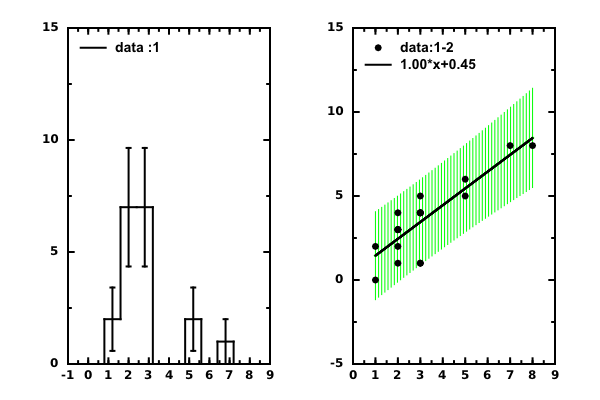## Linear regression with HFitter

Now we can do a bit more flexible fit defining a linear function analytically. We will usejhplot.HFitter which allows to define any fit function. We simulate a linear dependence of the variable Y on X using random numbers: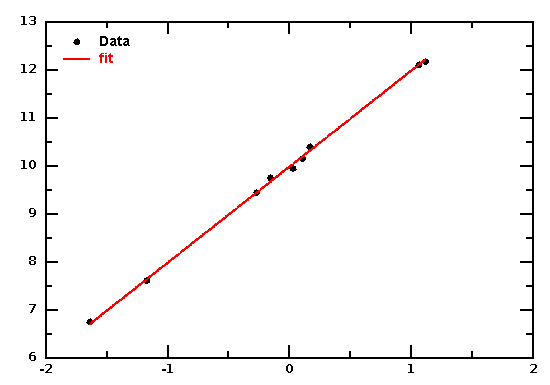The fit returns exactly the same a and b values which were used to generate data:

a = 9.99526486454, b = 1.99636040719

## Non-linear fitting

Data fits can be done by using the Java classjhplot.HFitter. But before, one needs to construct a function and then set initial values for free parameters. Fitting can be done either in the interactive mode or using a script.

By default, HFitter fits data using chi2 methods. It is important to specify errors on Y-values of input data. You can print the available methods as:

from jhplot import *
f=HFitter()
print f.getFitMethod()

which prints "leastsquares", "cleverchi2", "chi2", "bml" fitting methods. You can set the fit method when initialize the fitter:

from jhplot import *
f=HFitter("leastsquares")

In this case, instead "chi2", "leastsquares" fit method is used. In this case, you can fit "X-Y" data arrays without specifying errors on Y.

Let's make a simple chi2 fit of experimental data using an analytic function $\displaystyle{ Tu + (Ta - Tu) * exp(-kk * x) }$, where parameters Tu, Ta and kk need to be determined by fitting data stored as array. The data has experimental (statistical or systematic) uncertainties. This is mandatory for chi2 minimization. The resulting fit is shown below (example is provided by Klaus Rohe).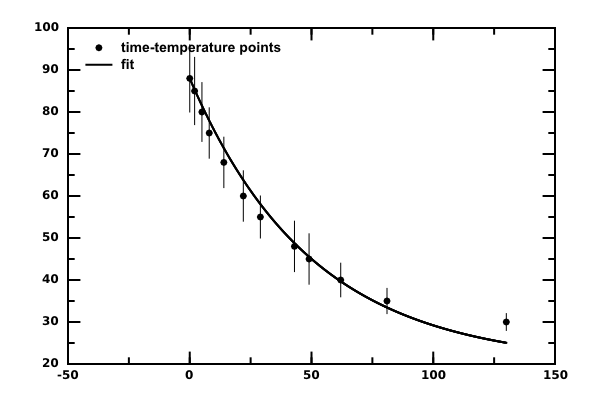This image is generated by the code given below where we usejhplot.P1D container to store input data. You can access fit errors and the fit quality (chi2/ndf) as described by thejhplot.HFitter class.

Below we will illustrate how to perform a rather complicated fit using the chi2 method. The fit will be done in several steps. In this example we fit data which can be described by multiple Gaussians, in which next Gaussians fit takes the values from the previous fit:

The output is shown here: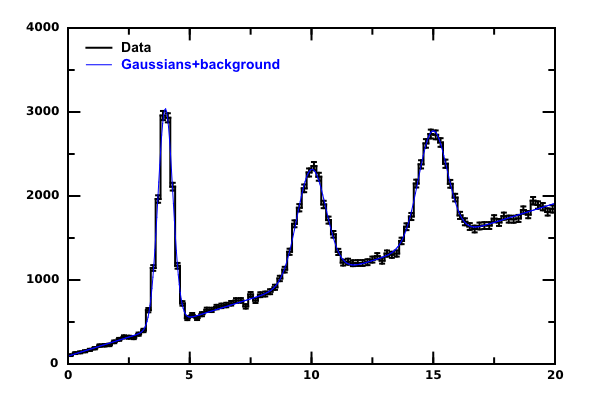Not allowed to read See [https://datamelt.org/code/index.php?keyword=hfitter HFitter code examples] : Not datamelt.org URL!

## Numerical interpolation

One can use numerical way to describe data using Interpolation and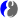Smoothing One example is shown on this figure: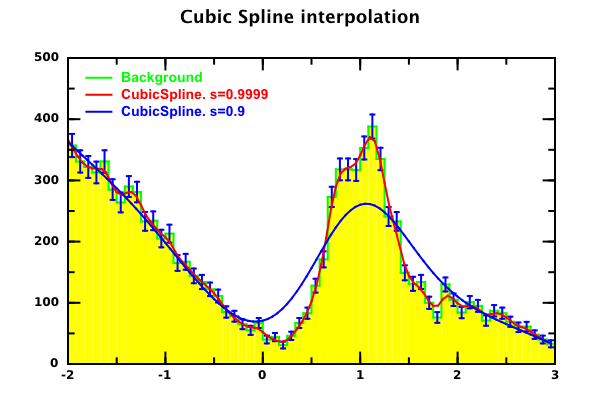where we attempted to smooth data using a non-analytical approach. Such approach is often considered for various predictions when to find an appropriate analytical function is difficult or impossible. DataMelt provides a several flexible methods to smooth data and perform interpolation. The code of this example is given below:

## Interactive fit

One simple way to fit data using interactive fitter is to use the classjhplot.HFit. This fitter creates a dialog that is shown in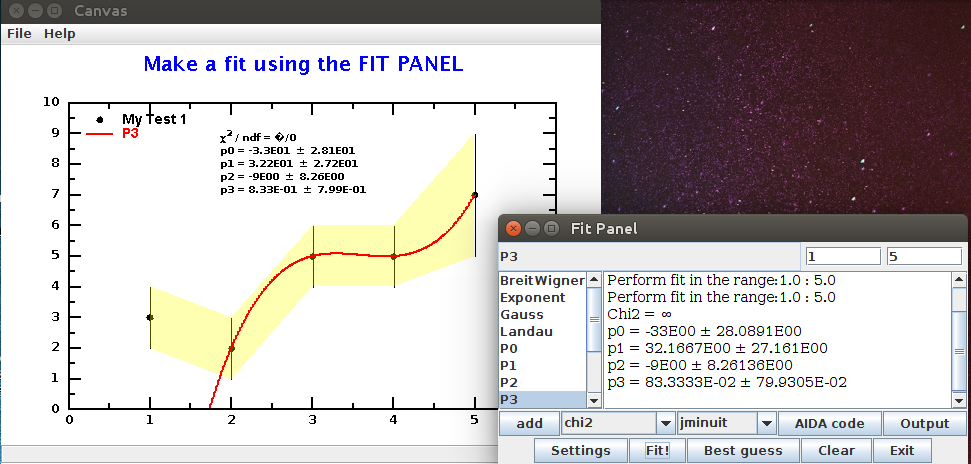The code that creates this dialog window is shown here:

Data can be fitted using many predefined functions in an more interactive way. Let's create a few data containers (1D array, 1D histograms) and startjhplot.HPlotJas plotter based on JAS2 program from Slac:

from java.util import Random
from jhplot  import *

h1 = H1D("1D  histogram",100, -2, 2.0)
p1=P1D("data with errors")

rand = Random()
for i in range(600):
h1.fill(rand.nextGaussian())
p0=P0D("Normal distribution")
p0.randomNormal(1000,0.0,10.0)
from  java.util import ArrayList
a=ArrayList([h1,p1,p0])
c=HPlotJas("JAS",ArrayList([h1,p1,p0]))

This will bring up a "JAS" program with all objects shown on the left side.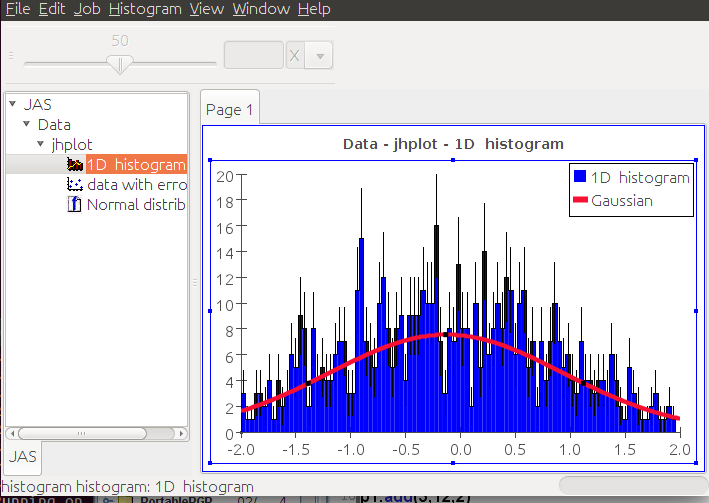You can expand the tree (left) and click on each object to plot on the canvas. Then try to fit the data: using the mouse pop-up dialog, press "Add function" (for example, a Gaussian function) and click on "Fit". The program will perform chi2 minimisation and you will see a Gaussian line on to of the data. You can adjust the initial values of the function by dragging 3 points of this function.

In the above example we used pre-built functions to perform fits. You can add your own custom fit function to the menu and use it for fitting as well. You can do this directly in the Jython script and pass this function to HplotJas canvas. Below we show an example in which we create 2 custom functions (a new Gaussian and a parabola) and passed them to the HPlotJas for interactive fitting.